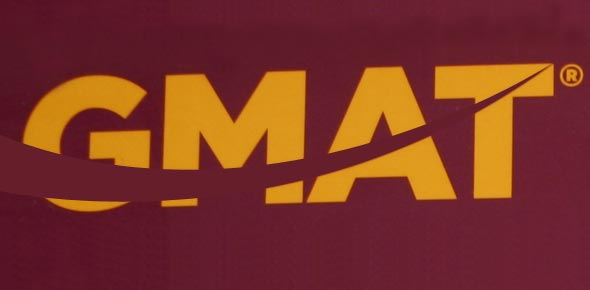# GMAT Memorization Trivia Test! Quiz Questions

113 Questions | Total Attempts: 136SettingsHow well do you think you will do in a GMAT memorization trivia test! There are different ways that the assessment can test your memorization ability and it is important to ensure that you have as much practice as you need before you go for the main test. This trivia questions will help prepare you, do give them a try and see how good you will do. All the best!

• 1.
12.5% = what fraction?
• 2.
16.66% = what fraction?
• 3.
37.5% = what fraction?
• 4.
62.5% = what fraction?
• 5.
66.66% = what fraction?
• 6.
83.33% = what fraction?
• 7.
87.5% = what fraction?
• 8.
1/8 = what percent?
• 9.
1/6 = what percent?
• 10.
3/8 = what percent?
• 11.
5/8 = what percent?
• 12.
5/6 = what percent?
• 13.
7/8 = what percent?
• 14.
112=?
• 15.
122=?
• 16.
132=?
• 17.
142=?
• 18.
152=?
• 19.
43=?
• 20.
53=?
• 21.
63=?
• 22.
73=?
• 23.
83=?
• 24.
93=?
• 25.
√2 ≈ ?
Related TopicsBack to top